# Projective plane

(diff) ← Older revision | Latest revision (diff) | Newer revision → (diff)

two-dimensional projective space

An incidence structure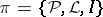(cf. Incidence system). The elements of the set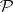are called points, the elements of the setare called (straight) lines andis an incidence relation. An incidence structure satisfies the following axioms:

1) for any two distinct pointsandthere is a unique linesuch that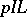and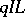;

2) for any two distinct linesandthere is a unique pointsuch thatand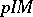; and

3) there exist four points no three of which are incident with one line.Figure: p075310a

For example, the setof lines and planes of three-dimensional affine space, passing through a point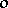, is a projective plane if as projective points one takes the lines ofand as projective lines one takes the planes of. In this interpretation the homogeneous coordinates of a point of the projective plane over a field have a clear geometric meaning as the coordinates of some vector on the line corresponding to the point (cf. Projective geometry; Projective coordinates). Another example is the projective plane constituted by seven points,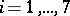, and the seven lines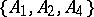,,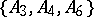,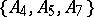,,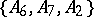,(Fig. a). It is a representative of the class of finite projective planes. A projective plane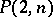is called a finite projective plane of orderif the incidence relation satisfies one more axiom:

4) there is a line incident with exactlypoints.

Inevery point (line) is incident withlines (points), and the number of points of the plane, which is equal to the number of lines, is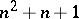. The question for which values ofa projective planeexists is unanswered (1990). The existence of a finite projective plane whose order is a power of a prime number has been proved (cf. ). The non-existence ofhas been proved for a large class of numbers: Ifis congruent to 1 or 2 modulo 4 and if in the prime factorization ofthere is at least one prime number congruent to 3 modulo 4 that occurs with odd exponent, thendoes not exist. Such are, e.g.,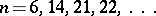. The problems remain open for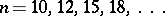. An important problem in the theory of finite projective planes is the study of subplanes of a given. E.g., if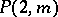is a proper subplane of, then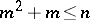or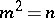(cf. ).

The concept of duality is characteristic for projective planes. Two projective planes are said to be dual if between the points (lines) of one plane and the lines (points) of the other there is a one-to-one correspondence preserving incidence. Certain projective planes (e.g. projective planes over a field) admit a dual mapping onto itself, called a polarity; projective planes admitting a polarity are called self-dual. The so-called little duality principle holds for projective planes: If some statementabout points and lines of a projective plane formulated solely in terms of incidence between them holds, then the statementdual toalso holds, i.e. the statement obtained fromby replacing the word "point" with "line" and vice versa also holds.

An isomorphic mapping of a projective plane onto itself is called a collineation. A collineation of a finite projective planeis a permutation of the set of points and a permutation of the set of lines, where these two permutations are compatible. A finite projective plane is Desarguesian if it has a group of collineations that acts doubly-transitively on its points. The group of collineations of a Desarguesian projective plane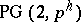has order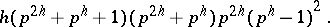The group of collineations of a non-Desarguesian projective planehas order at most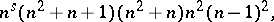where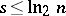. The orders of the groups of collineations of the known non-Desarguesian projective planes do not exceed the orders of the groups of collineations of the Desarguesian planes of the same order.

The Lenz–Barlotti classification of projective planes is based on considering the 53 types of setsdefined for full collineation groups. One of the basic approaches to study projective planes is the introduction of coordinates and a ternary operation in these planes. To every possible type of projective plane in the Lenz–Barlotti classification corresponds a system of algebraic laws that must be satisfied by the natural coordinate domain of the projective planes determined by the ternary operation. E.g., a projective plane is Desarguesian (Pappian) if and only if all its natural coordinate domains are skew-fields (fields). A Desarguesian finite projective planeis Pappian.

A special feature of a finite Desarguesian projective planeis that it has a collineation of orderthat acts cyclically on points and lines. This makes it possible to representby a cyclic array. This representation ofis as follows. Its points, enumerated by the natural numbers from 1 to, are distributed over a rectangular array ofrows andcolumns such that each column, which represents a line with all points on it, is obtained by adding 1 (modulo) to the elements of the previous column. E.g., a representation ofis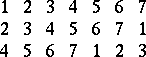The planes,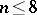, are unique up to an isomorphism — they are Desarguesian or Galois planes (cf. ), while already for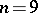four non-isomorphic planes are known (cf. ).

If to the axioms of a projective plane and Desargues' assumption one adds order axioms (described by separation of pairs of points lying on one straight line, e.g. in Fig. b the pairseparates the pair, while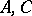does not separate) and the continuity axiom, then the projective plane thus obtained is isomorphic to the real affine plane completed by improper elements: an improper (infinitely-distant) point is added to each line, to parallel lines the same point, to non-parallel lines distinct points, and the improper points are required to ly on one improper straight line.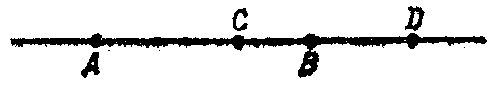Figure: p075310b

A projective plane is called topological if the sets of its points and lines are topological spaces and if the join and intersection are continuous. In a topological plane the ternary operation is continuous in all its arguments. From the topological point of view the point set of a real projective plane (as well as the set of lines) is a closed non-orientable manifold whose Euler characteristic is 1.

How to Cite This Entry:
Projective plane. Encyclopedia of Mathematics. URL: http://encyclopediaofmath.org/index.php?title=Projective_plane&oldid=14467
This article was adapted from an original article by V.V. Afanas'ev (originator), which appeared in Encyclopedia of Mathematics - ISBN 1402006098. See original article